# SAT Math Multiple Choice Question 818: Answer and Explanation

### Test Information

Question: 818

8. A math teacher decides to create several practice tests for her students before theytake the SAT. She wants to make some non-calculator tests and some calculator testsso that her students will be able to practice both. She figures that each non-calculatortest will take her 3 hours to create, and each calculator test will take 4 hours tocreate. If she is willing to devote at most 6 hours per week of her time for the next5 weeks to create the practice tests, and she wants to provide at least 8 practicetests, which of the following systems of inequalities can help her determine how manyof each type of test she can create?

• A.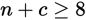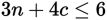• B.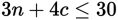• C.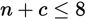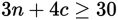• D.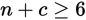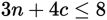Explanation:

B

Difficulty: Medium

Category: Heart of Algebra / Inequalities

Strategic Advice: Use the Kaplan Strategy for Translating English into Math. The clue "at least" means that many or more, so use the symbol ≥ when describing the number of tests. The clue "at most" means that many or less, so use the symbol ≤ when describing the number of hours.

Getting to the Answer: First assign variables: Let n represent the number of non-calculator tests and c represent the number of calculator tests. Write an inequality to describe the number of tests:

non-calculator plus calculator is at least 8: n + c ≥ 8

You can already eliminate C and D. Next, write an inequality to describe the number of hours needed to make the tests. Be sure to incorporate all 5 weeks of time the teacher is willing to devote:

hours per non-calc. test times # of tests: 3n plus hours per calc. test times # of tests: + 4c is at most 5 hours per week times 6 weeks: ≤ 30Put the two inequalities together to form the system given in (B).